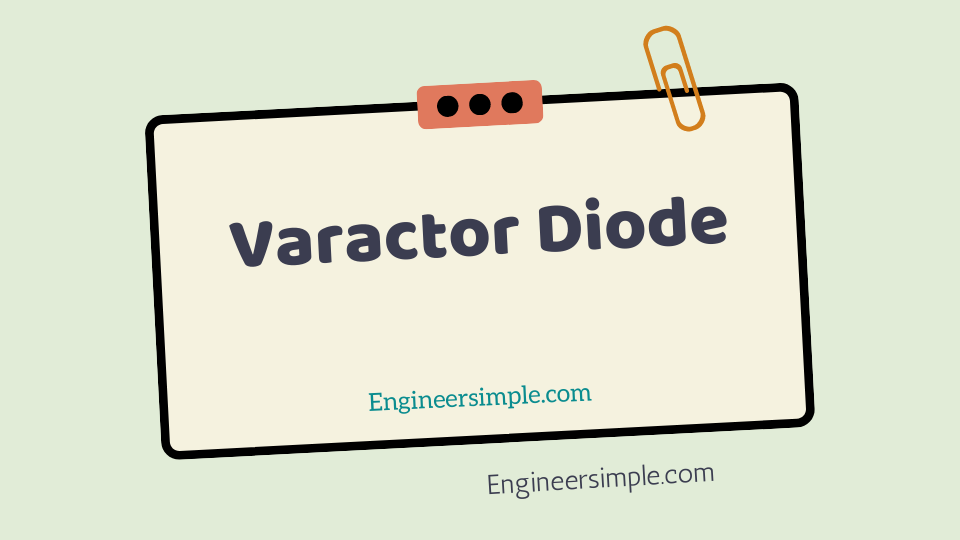Varactor Diode - Engineer Simple

#A junction diode which acts as a variable capacitor under changing reverse bias is known as avaractor diode.When a pn junction is formed, depletion layer is created in the junction area. Since there are nocharge carriers within the depletion zone, the zone acts as an insulator. varactor diode application The p-type material with holes(considered positive) as majority carriers and n-type material with electrons (−ve charge) as majoritycarriers act as charged plates. Thus the diode may be consider as a capacitor with n-region and p-region forming oppositely charged varactor diode application plates and with depletion zone between them acting as a dielec-tric. This is illustrate in Fig.1 (i).

A varactor diode is specially construct to have high capaci-tance under reverse bias. Fig.1 (ii) shows the symbol of varactor diode. The values of capaci-tance of varactor diodes are in the picofarad (10−12 F) range.

## Theory

For normal operation, a varactor diode is always*reverse bias.

The capacitance ofvaractor diode is find as :

where,

CT= Total capacitance of the junction;

ε= Permittivity of the semiconductormaterial;

A= Cross-sectional area of the junction;

Wd= Width of the depletion layer.

When reverse voltage across a varactor diode isincreased, the width Wd of the depletion layer increases. Therefore, the total junction capacitance CT of thejunction decreases. On the other hand, if the reversevoltage across the diode is lowered, the width Wd of thedepletion layer decreases. Consequently, the total junctioncapacitance CT increases.*A forward biased varactor diode would serve no useful purpose.